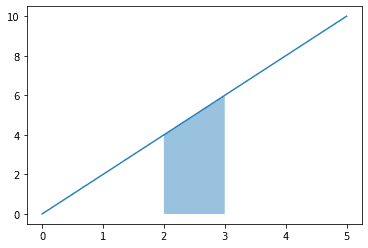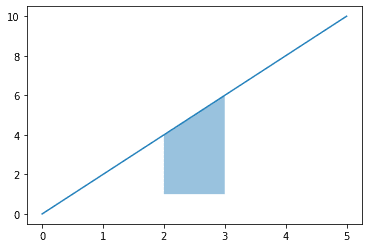search
Search
menu search toc more_vert
Guest 0reps
Thanks for the thanks!
close
account_circle
Profile
exit_to_app
Sign out
help Ask a question
search
keyboard_voice
close
Searching Tips
Search for a recipe:
"Creating a table in MySQL"
Search for an API documentation: "@append"
Search for code: "!dataframe"
Apply a tag filter: "#python"
Useful Shortcuts
/ to open search panel
Esc to close search panel
to navigate between search results
d to clear all current filters
Enter to expand content previewDoc SearchCode Search BetaSORRY NOTHING FOUND!
mic
Start speaking...Voice search is only supported in Safari and Chrome.
Shrink
Navigate to
A
A
brightness_medium
share
arrow_backShareTwitterFacebook
0
thumb_down
0
chat_bubble_outline
0
auto_stories new
settings

# Filling in the area underneath a curve in Matplotlib

Matplotlib
chevron_right
Cookbooks
chevron_right
Colors Cookbook
schedule Jul 1, 2022
Last updated
local_offer PythonMatplotlib
Tags
expand_more

To fill the area underneath a curve in Matplotlib, use the `plt.fill_between(~)` method:

``` import numpy as npimport matplotlib.pyplot as pltx = np.linspace(0, 5, 1000)plt.plot(x, 2*x)# Fill from x=2 to x=4x_fill = np.linspace(2, 3, 1000)plt.fill_between(x_fill, 2*x_fill, alpha=0.5) # suppose f(x)=2xplt.show() ```

The three arguments that we've supplied to `fill_between(~)` are as follows:

• the x-values to shade

• the upper bound of the y-values to shade in. Note that the lower bound is set to 0 by default.

• the transparency of the shaded area.

This gives us the following output:# Adjusting the baseline

By default, the lower bound is fixed at y=0. We can set a custom baseline by supplying one additional argument:

``` import numpy as npimport matplotlib.pyplot as pltx = np.linspace(0, 5, 1000)plt.plot(x, 2*x)# Fill from x=2 to x=3x_fill = np.linspace(2, 3, 1000)plt.fill_between(x_fill, 1, y2=2*x_fill, alpha=0.5) # suppose f(x)=2xplt.show() ```

Here, the second argument is our new baseline `y=1`, and the upper bound is set to the line.

The output is as follows:mail